# Cylinder Venn Diagram

You need design Cylinder Venn Diagram? Nothing could be easier with ConceptDraw DIAGRAM diagramming and vector drawing software extended with Venn Diagrams Solution from the â€śDiagramsâ€ť Area. ConceptDraw DIAGRAM allows you to design various Venn Diagrams including Cylinder Venn Diagrams.

Venn Diagrams Solution provides you the tools for quick and easy creating Venn Diagrams:

• Venn Diagrams library with great quantity of predesigned vector objects;
• Large set of templates and samples that you can use as the base designing your own Venn Diagrams.

Use ConceptDraw STORE to get quickly the access to the Venn Diagrams templates and samples.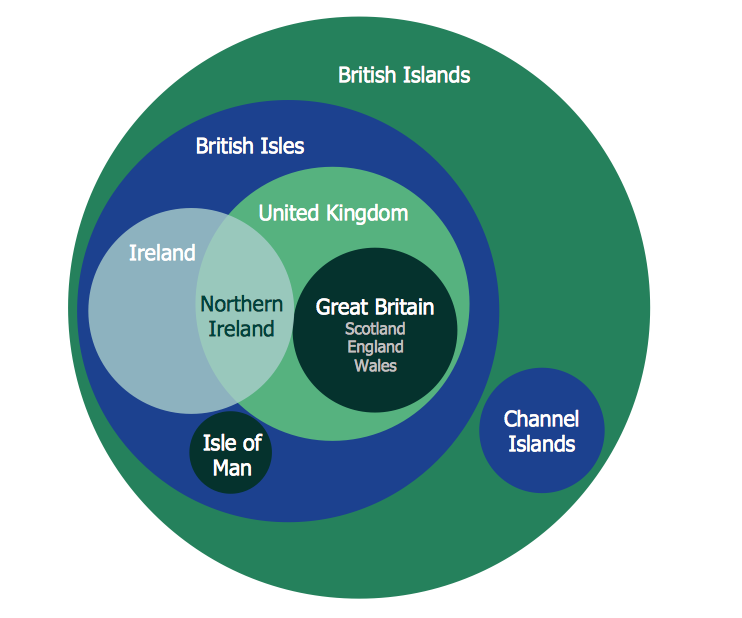Example 1. Cylinder Venn Diagram

This sample was created in ConceptDraw DIAGRAM using the Venn Diagrams Solution from the â€śDiagramsâ€ť Area and shows the British Islands Venn Diagram. An experienced user spent 5 minutes creating this sample.

Use the Venn Diagrams Solution for ConceptDraw DIAGRAM to create your own Cylinder Venn Diagrams of any complexity quick, easy and effective.

The Venn Diagrams designed with ConceptDraw DIAGRAM are vector graphic documents and are available for reviewing, modifying, converting to a variety of formats (image, HTML, PDF file, MS PowerPoint Presentation, Adobe Flash or MS Visio), printing and send via e-mail in one moment.

Related Solution:

### Venn Diagram Examples for Problem Solving.Venn Diagram as a Truth Table

Venn diagrams are illustrations used in the branch of mathematics known as set theory. They show the mathematical or logical relationship between different groups of things (sets). A Venn diagram shows all the possible logical relations between the sets.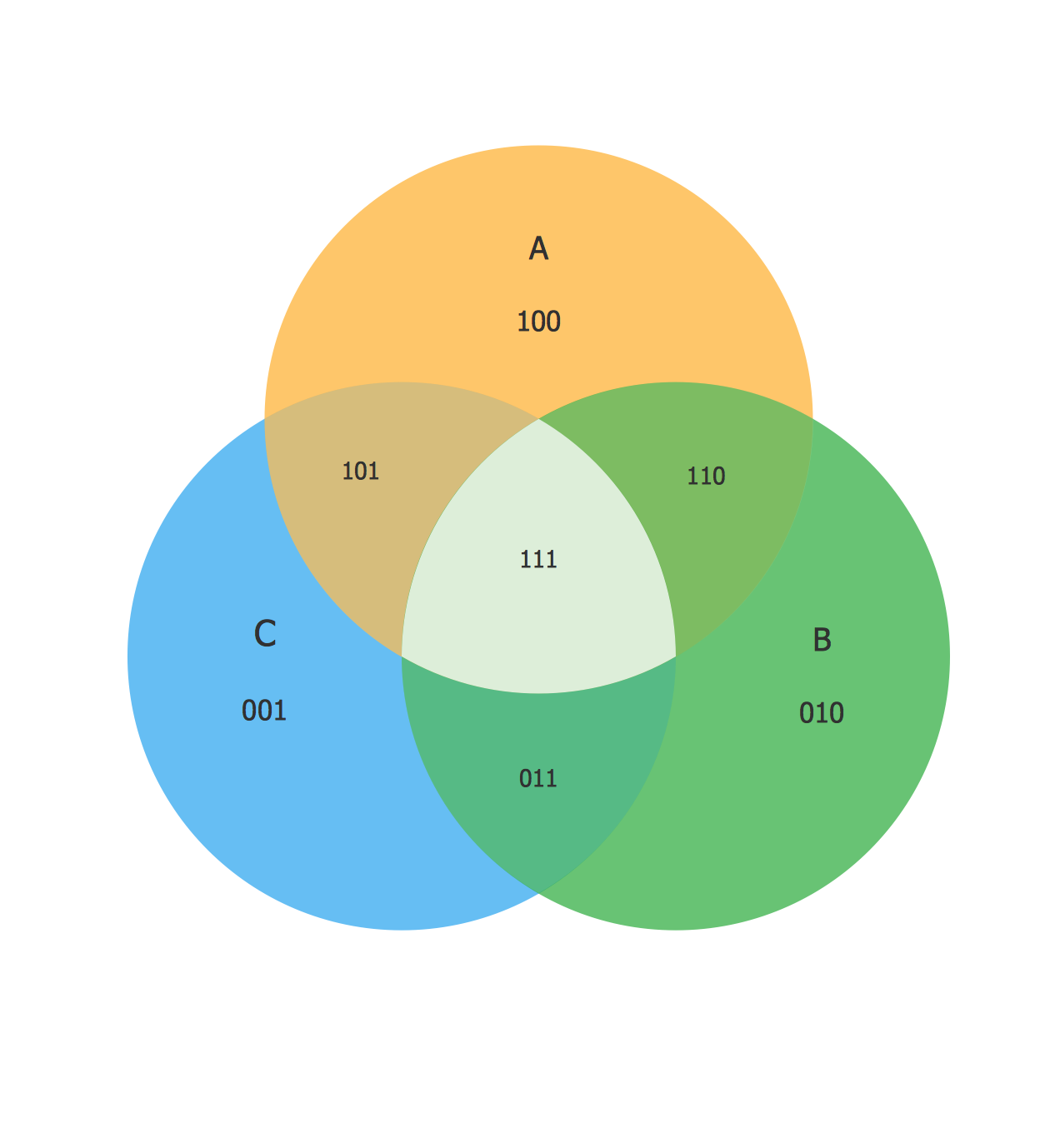Picture: Venn Diagram Examples for Problem Solving.Venn Diagram as a Truth Table
Related Solution:

### Venn Diagram Examples for Problem Solving . Quantum Information Science. BQP Complexity

Venn diagrams are illustrations used in the branch of mathematics known as set theory. They show the mathematical or logical relationship between different groups of things (sets). A Venn diagram shows all the possible logical relations between the sets.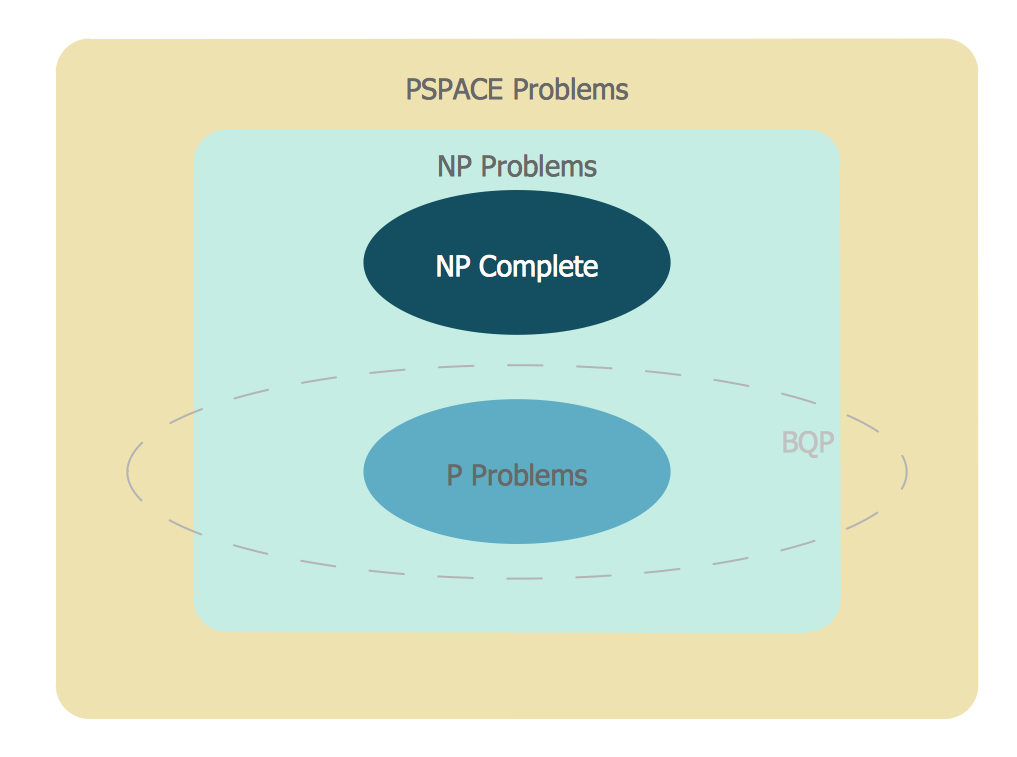Picture: Venn Diagram Examples for Problem Solving . Quantum Information Science. BQP Complexity
Related Solution:

### Venn Diagram

Venn diagrams are illustrations used in the branch of mathematics known as set theory. They show the mathematical or logical relationship between different groups of things (sets). A Venn diagram shows all the possible logical relations between the sets.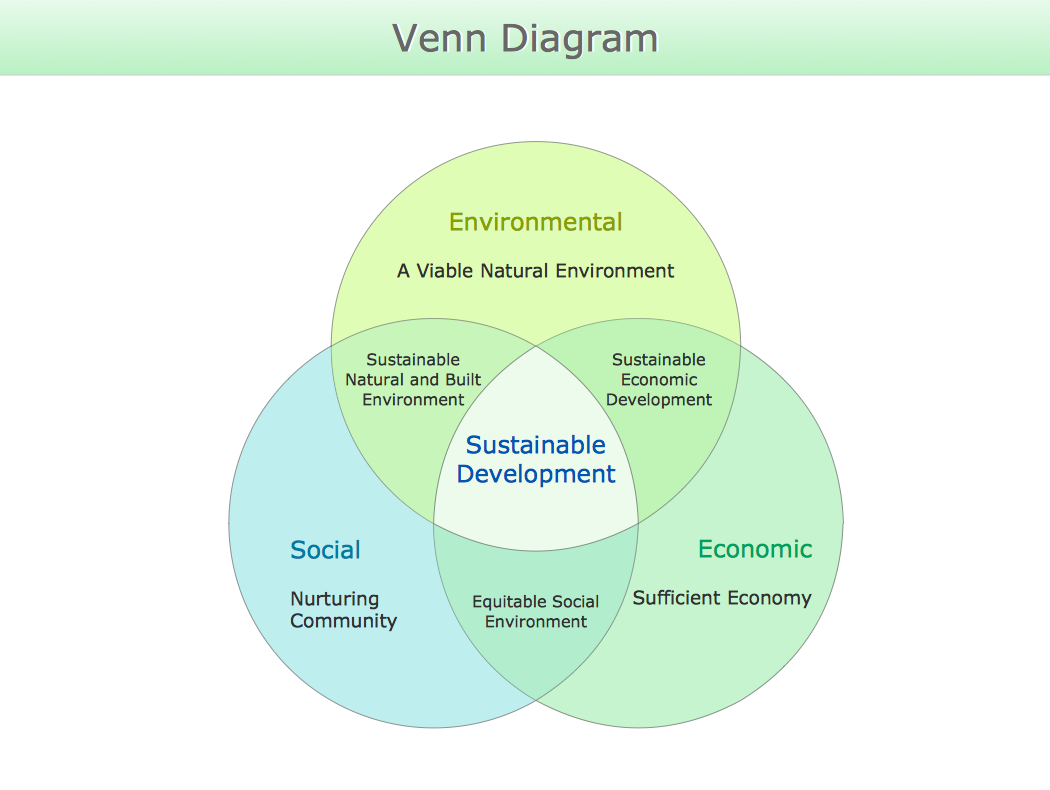Picture: Venn Diagram
Related Solution:

### Basic Flowchart Symbols and Meaning

While creating flowcharts and process flow diagrams, you should use special objects to define different statements, so anyone aware of flowcharts can get your scheme right. There is a short and an extended list of basic flowchart symbols and their meaning. Basic flowchart symbols include terminator objects, rectangles for describing steps of a process, diamonds representing appearing conditions and questions and parallelograms to show incoming data. This diagram gives a general review of the standard symbols that are used when creating flowcharts and process flow diagrams. The practice of using a set of standard flowchart symbols was admitted in order to make flowcharts and other process flow diagrams created by any person properly understandable by other people. The flowchart symbols depict different kinds of actions and phases in a process. The sequence of the actions, and the relationships between them are shown by special lines and arrows. There are a large number of flowchart symbols. Which of them can be used in the particular diagram depends on its type. For instance, some symbols used in data flow diagrams usually are not used in the process flowcharts. Business process system use exactly these flowchart symbols.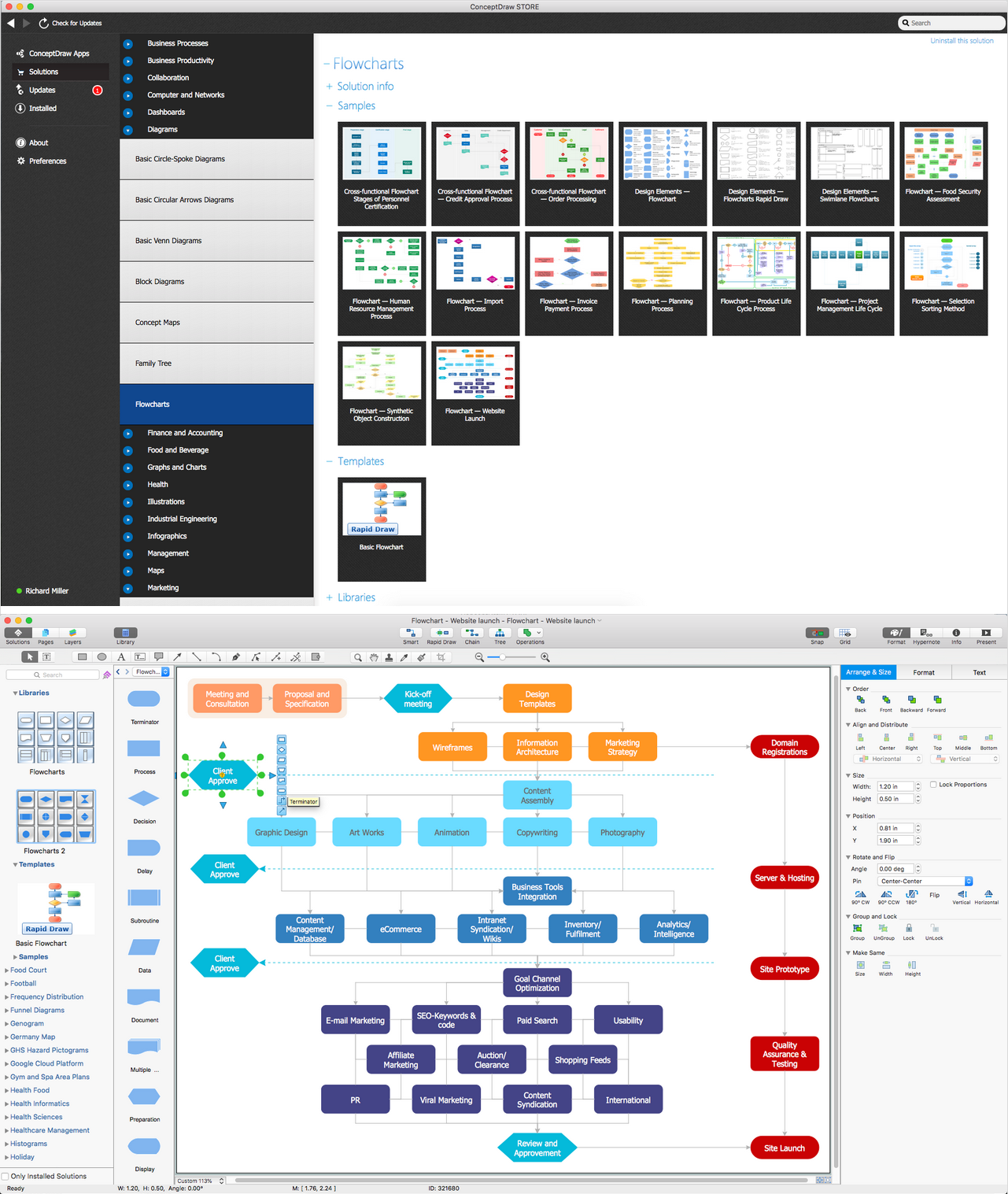Picture: Basic Flowchart Symbols and Meaning
Related Solution:

### 3 Circle Venn. Venn Diagram Example

This example shows the 3 Circle Venn Diagram. The Venn Diagrams visualize all possible logical intersections between several sets. On this example you can see the intersections of 3 sets. Venn Diagrams are widely used in mathematics, logic, statistics, marketing, sociology, etc.Picture: 3 Circle Venn. Venn Diagram Example
Related Solution:

### Circle Spoke Diagram Template

ConceptDraw DIAGRAM v12 diagramming and vector drawing software offers the Circle Spoke Diagram Template included in the Circle-Spoke Diagrams Solution from the "What is a Diagram" Area. Use of ready template is the easiest way of drawing professional looking Circle-Spoke Diagrams.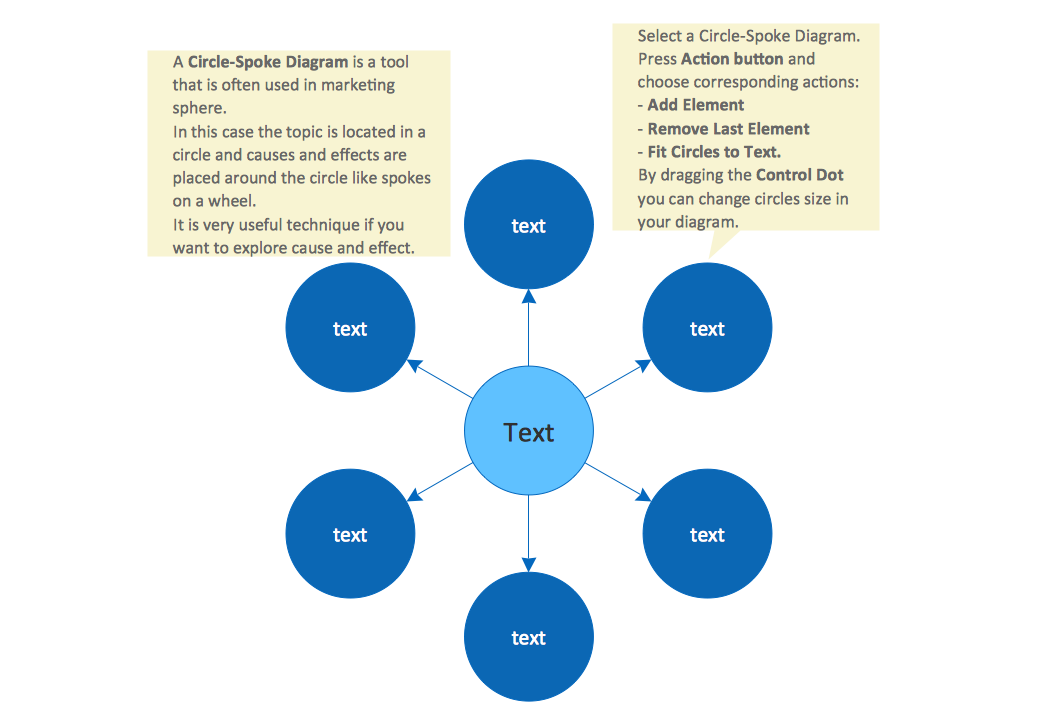Picture: Circle Spoke Diagram Template
Related Solution:

### 3 Circle Venn Diagram. Venn Diagram Example

This template shows the Venn Diagram. It was created in ConceptDraw DIAGRAM v12 diagramming and vector drawing software using the ready-to-use objects from the Venn Diagrams Solution from the "What is a Diagram" area of ConceptDraw Solution Park. Venn Diagrams visualize all possible logical intersections between several sets and are widely used in mathematics, logic, statistics, marketing, sociology, etc.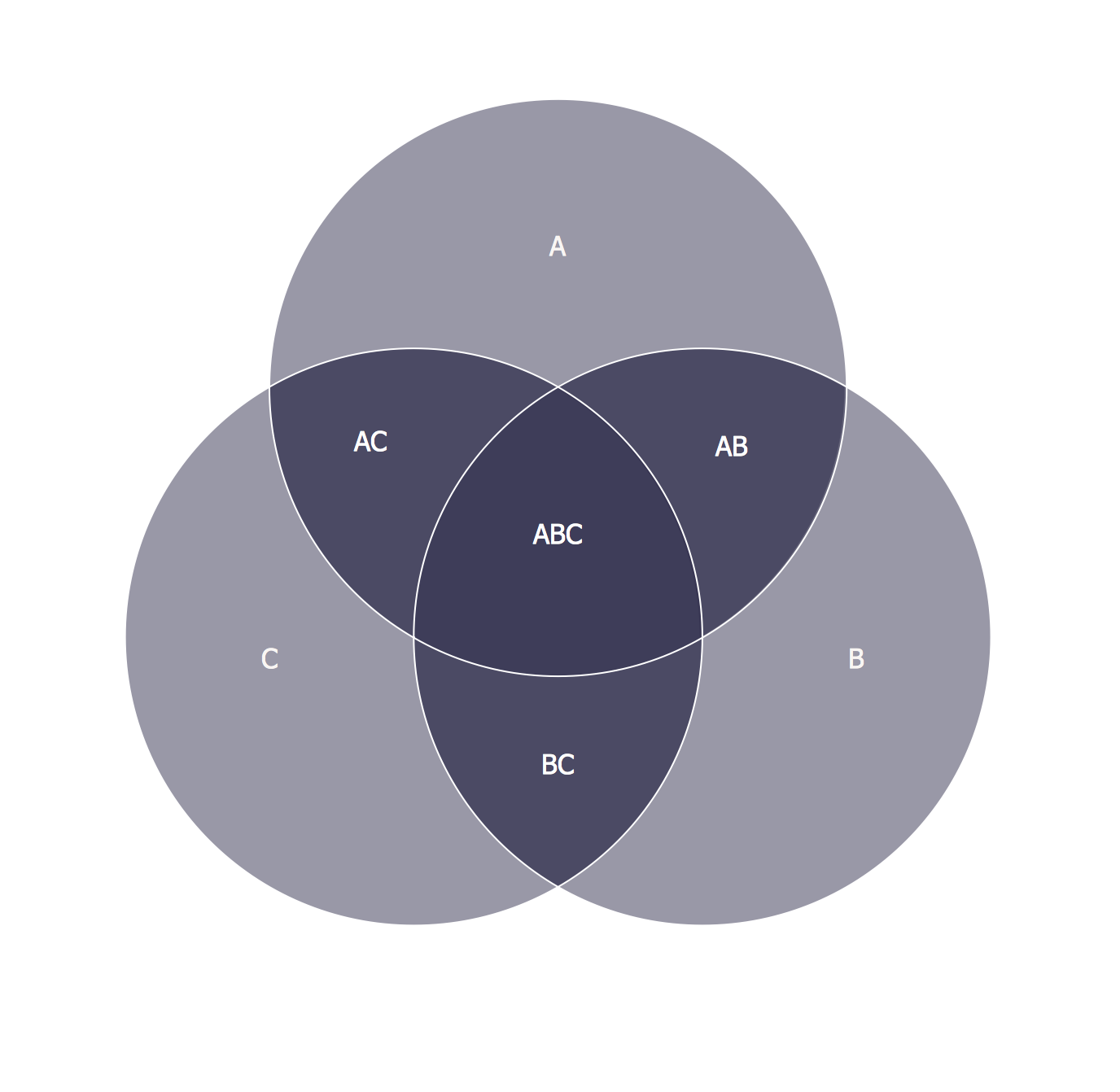Picture: 3 Circle Venn Diagram. Venn Diagram Example
Related Solution:

### Venn Diagram Examples for Problem Solving. Computer Science. Chomsky Hierarchy

A Venn diagram, sometimes referred to as a set diagram, is a diagramming style used to show all the possible logical relations between a finite amount of sets. In mathematical terms, a set is a collection of distinct objects gathered together into a group, which can then itself be termed as a single object. Venn diagrams represent these objects on a page as circles or ellipses, and their placement in relation to each other describes the relationships between them. The Venn diagram example below visualizes the the class of language inclusions described by the Chomsky hierarchy.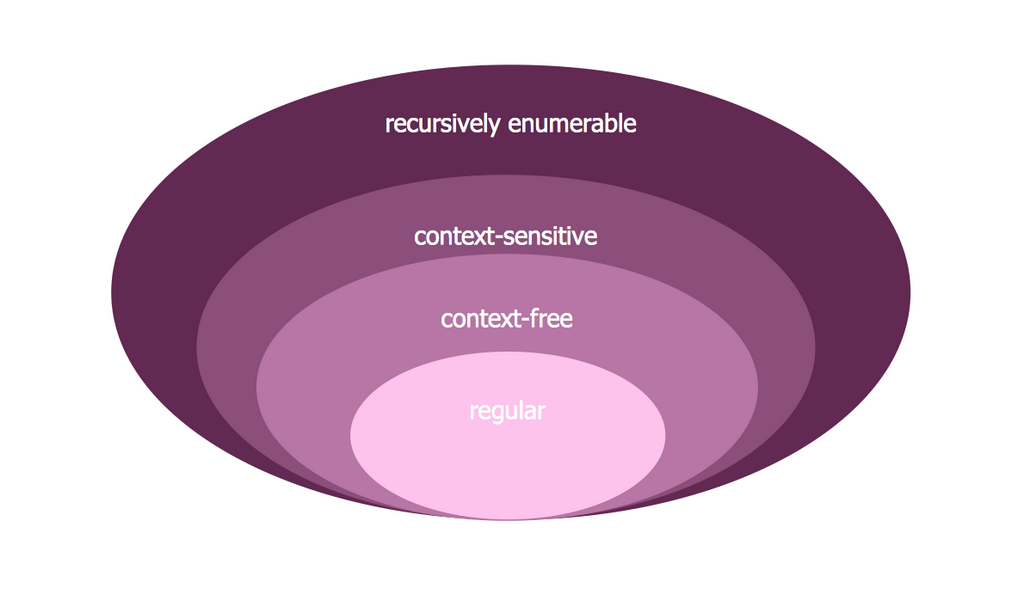Picture: Venn Diagram Examples for Problem Solving. Computer Science. Chomsky Hierarchy
Related Solution:

### Multi Layer Venn Diagram. Venn Diagram Example

To visualize the relationships between subsets of the universal set you can use Venn diagrams. To construct one, you should divide the plane into a number of cells using n figures. Each figure in the chart represents a single set of, and n is the number of represented sets. Splitting is done in a way that there is one and only one cell for any set of these figures, the points of which belong to all the figures from the set and do not belong to others. The plane on which the figures are represented, is the universal set U. Thus, the point which does not belong to any of the figures, belongs only to U.Picture: Multi Layer Venn Diagram. Venn Diagram Example
Related Solution:

### Concept Maps

As concept map consists from conception-blocks and links between them, you can use ConceptDraw library objects and add to a document as much objects as you need. ConceptDraw tools let you to edit, group, move objects, change their appearance and add text signs and raster or vector pictures. ConceptDraw DIAGRAM v12 diagramming and vector drawing software extended with Concept Maps Solution from the "What is a Diagram" Area is effective tool for creating professional looking Concept Maps.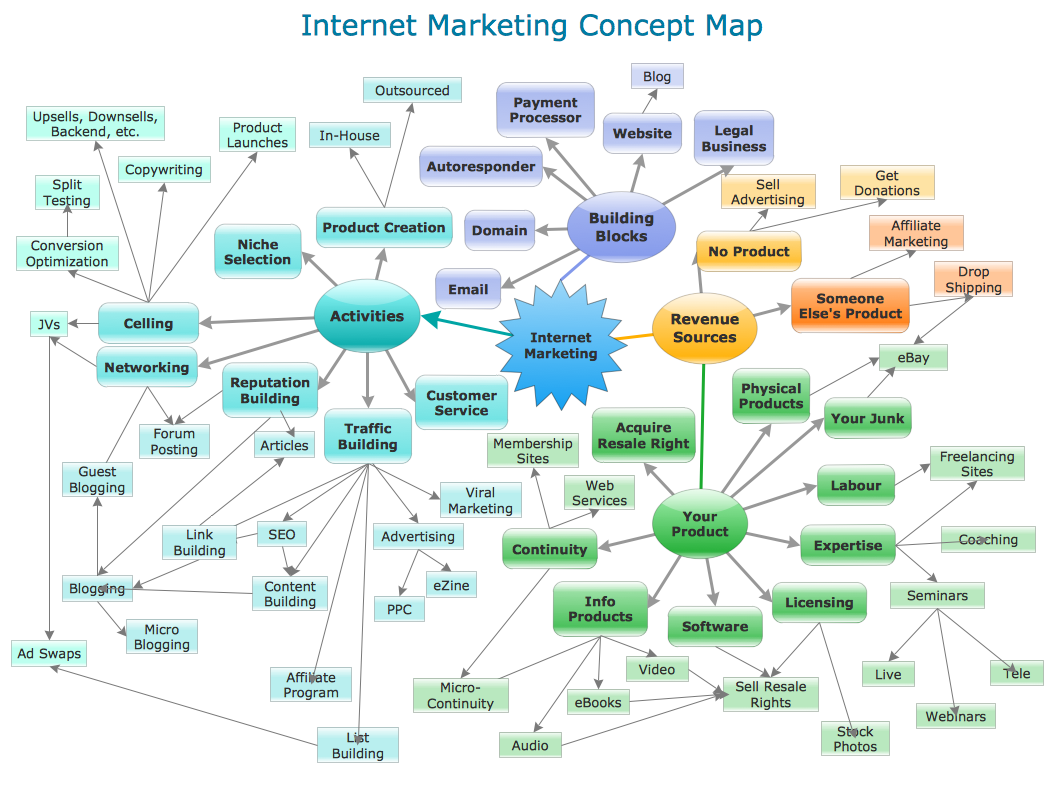Picture: Concept Maps
Related Solution: